Courses

# Test: Solid Mechanics- 5

## 10 Questions MCQ Test GATE Civil Engineering (CE) 2022 Mock Test Series | Test: Solid Mechanics- 5

Description
This mock test of Test: Solid Mechanics- 5 for Civil Engineering (CE) helps you for every Civil Engineering (CE) entrance exam. This contains 10 Multiple Choice Questions for Civil Engineering (CE) Test: Solid Mechanics- 5 (mcq) to study with solutions a complete question bank. The solved questions answers in this Test: Solid Mechanics- 5 quiz give you a good mix of easy questions and tough questions. Civil Engineering (CE) students definitely take this Test: Solid Mechanics- 5 exercise for a better result in the exam. You can find other Test: Solid Mechanics- 5 extra questions, long questions & short questions for Civil Engineering (CE) on EduRev as well by searching above.
QUESTION: 1

### Shear center of the T-section can be shown by point S and center of gravity by point G. The correct representation of S and G for the section is :

Solution:

Shear centre is the point from which if a concentrated load passes then, there will be only bending and no twisting. It is also called centre of flexure. It is that point through which resultant of shear passes.

The shear centre (S) and centre of gravity (G) of some sections is shown below.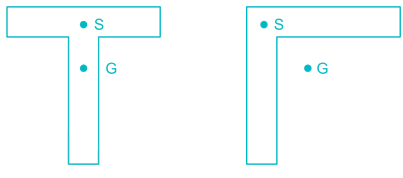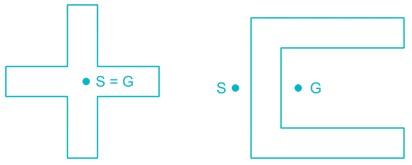QUESTION: 2

### Two equal length beams are fixed at their ends. One carries a distributed load and other carries same load but concentrated in the middle. The ratio of maximum deflections will be

Solution: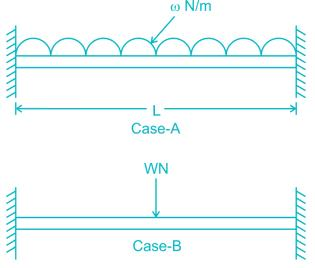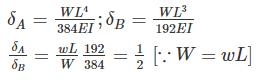QUESTION: 3

### A composite section made of two materials has moduli of elasticity in the ratio 1 : 2 and lengths in the ratio 2 : 1. The ratio of the corresponding deflection under a direct load is

Solution: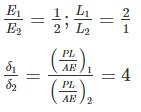QUESTION: 4

A cantilever beam of span L and uniform flexular rigidity ‘EI’ is loaded with a downward U.D.L of w kN/m and upward force ‘P’ at the free end. The deflection at the free end will be zero, if

Solution: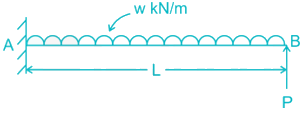Using Principal of superposition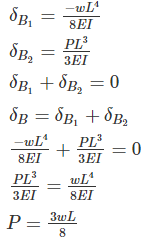QUESTION: 5

If the depth of a simply supported beam carrying an isolated load at its center, is doubled the deflection of the beam at the center will be changed by a factor of

Solution: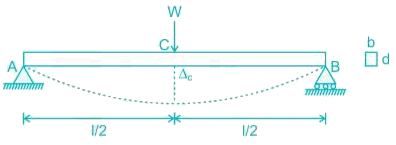The central deflection in a simply supported beam due to point load,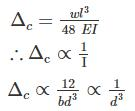As the depth of the beam is double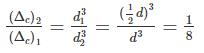QUESTION: 6

A force P is applied at a distance x from the end of the beam as shown in the figure. What would be the value of x so that the displacement at ‘A’ is equal to zero?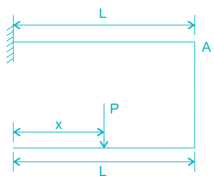Solution:

Load at point A is shown in figure below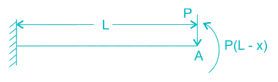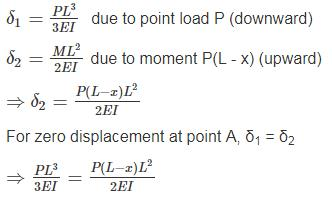⇒ x = L/3 = 0.33 L

QUESTION: 7

If the deflection at free end of a uniformly loaded cantilever beam is 18 mm and the slope of the deflection curve at the free end is 0.02 then the length of the beam is

Solution: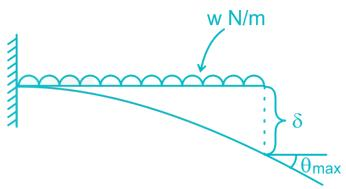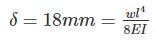_______1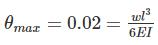_______2
From (2) ⇒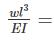0.02 × 6 = 0.12
substitute in equation (1)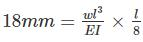⇒ l = 1.2 m

QUESTION: 8

A beam 40mm wide, 80mm deep, is freely supported over a span of 2m. A weight of 50 N is dropped on to the middle of the beam from a height of 40mm. Calculate the maximum instantaneous stress and deflection. E=200 GPa.

Solution:

Let W be equivalent static load that will produce same deflection as dynamic load applied from a height of 40 mm. Strain energy in beam due to dropping of 50 N load = 50 (40 + δ)

Strain energy in the beam due to load W applied gradually = ½ Wδ

50(40 + δ) = ½ Wδ                                            (1)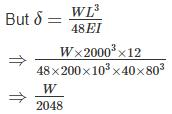Substituting in (1)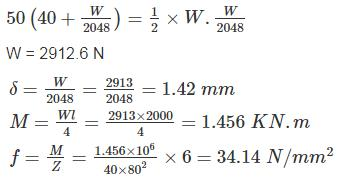*Answer can only contain numeric values
QUESTION: 9

Locate the shear center for the channel section as shown in the figure in mm?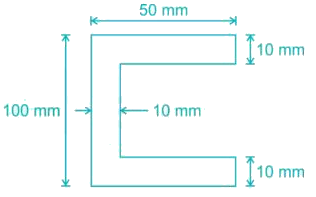Solution: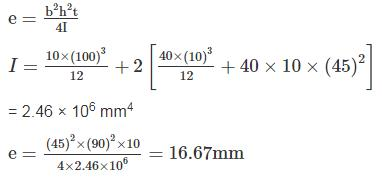QUESTION: 10

The equation for the deflected shape of a beam carrying a uniformly distributed load and simply supported at the ends is given by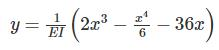What is the intensity of uniformly distributed load (in kN/m)? (The unit of EI is kN-m2 & it is a flexural rigidity)

Solution: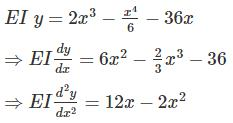But we know that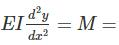(Bending moment atany section)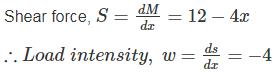⇒ w = 4 kN/m (Numerically)